# WO2016175342A2 - Ocean meteorological data sampling method performed by computer - Google Patents

## Info

Publication number
WO2016175342A2
WO2016175342A2 PCT/KR2015/004194 KR2015004194W WO2016175342A2 WO 2016175342 A2 WO2016175342 A2 WO 2016175342A2 KR 2015004194 W KR2015004194 W KR 2015004194W WO 2016175342 A2 WO2016175342 A2 WO 2016175342A2
Authority
WO
WIPO (PCT)
Prior art keywords
data
meteorological
number
sampling
zone
Prior art date
Application number
PCT/KR2015/004194
Other languages
French (fr)
Korean (ko)
Other versions
WO2016175342A3 (en
Inventor
김윤식
김광수
Original Assignee
한국해양과학기술원
Priority date (The priority date is an assumption and is not a legal conclusion. Google has not performed a legal analysis and makes no representation as to the accuracy of the date listed.)
Filing date
Publication date
Application filed by 한국해양과학기술원 filed Critical 한국해양과학기술원
Priority to PCT/KR2015/004194 priority Critical patent/WO2016175342A2/en
Publication of WO2016175342A2 publication Critical patent/WO2016175342A2/en
Publication of WO2016175342A3 publication Critical patent/WO2016175342A3/en

• 238000005070 sampling Methods 0 abstract claims description title 38
• 238000009826 distribution Methods 0 abstract claims description 37
• 230000001186 cumulative Effects 0 abstract claims description 12
• 238000005315 distribution function Methods 0 abstract claims description 10
• 238000000513 principal component analysis Methods 0 abstract claims description 6
• 238000004458 analytical methods Methods 0 abstract description 34
• 238000000034 methods Methods 0 claims description 20
• 239000007789 gases Substances 0 claims description 17
• 239000003570 air Substances 0 claims description 16
• 239000000284 extracts Substances 0 claims description 7
• 239000000047 products Substances 0 claims description 3
• 238000009740 moulding (composite fabrication) Methods 0 claims description 2
• 229910001868 water Inorganic materials 0 abstract 1
• 230000000875 corresponding Effects 0 description 12
• 238000000605 extraction Methods 0 description 9
• 230000002829 reduced Effects 0 description 4
• 230000014509 gene expression Effects 0 description 3
• 238000007405 data analysis Methods 0 description 2
• 230000001965 increased Effects 0 description 2
• 230000001603 reducing Effects 0 description 2
• 230000035945 sensitivity Effects 0 description 2
• 230000004075 alteration Effects 0 description 1
• 238000004364 calculation methods Methods 0 description 1
• 230000001721 combination Effects 0 description 1
• 230000002596 correlated Effects 0 description 1
• 238000007689 inspection Methods 0 description 1
• 239000000463 materials Substances 0 description 1
• 239000002609 media Substances 0 description 1
• 239000000203 mixtures Substances 0 description 1
• 238000006011 modification Methods 0 description 1
• 230000004048 modification Effects 0 description 1
• 230000004224 protection Effects 0 description 1
• 238000003908 quality control methods Methods 0 description 1
• 238000006467 substitution reaction Methods 0 description 1

## Images

•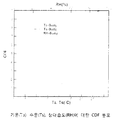•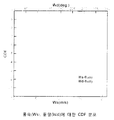•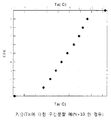•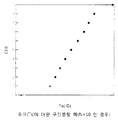•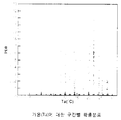•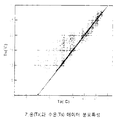•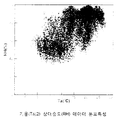•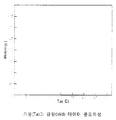•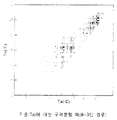•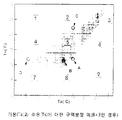•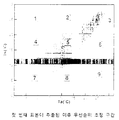••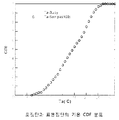•••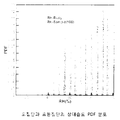••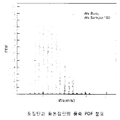•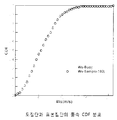•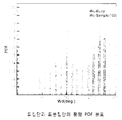•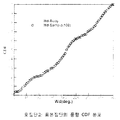•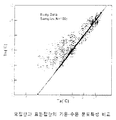•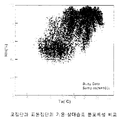•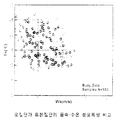••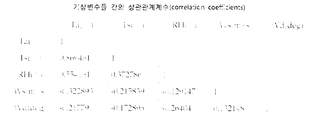••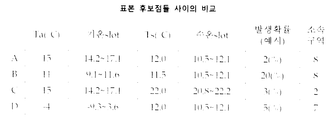•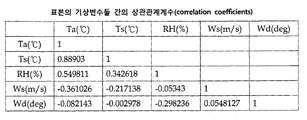## Classifications

• GPHYSICS
• G01MEASURING; TESTING
• G01WMETEOROLOGY
• G01W1/00Meteorology
• G01W1/02Instruments for indicating weather conditions by measuring two or more variables, e.g. humidity, pressure, temperature, cloud cover, wind speed
• GPHYSICS
• G01MEASURING; TESTING
• G01WMETEOROLOGY
• G01W1/00Meteorology
• G01W1/10Devices for predicting weather conditions
• GPHYSICS
• G06COMPUTING; CALCULATING; COUNTING
• G06FELECTRIC DIGITAL DATA PROCESSING
• G06F17/00Digital computing or data processing equipment or methods, specially adapted for specific functions
• G06F17/10Complex mathematical operations
• G06F17/16Matrix or vector computation, e.g. matrix-matrix or matrix-vector multiplication, matrix factorization
• GPHYSICS
• G06COMPUTING; CALCULATING; COUNTING
• G06NCOMPUTER SYSTEMS BASED ON SPECIFIC COMPUTATIONAL MODELS
• G06N20/00Machine learning
• GPHYSICS
• G16INFORMATION AND COMMUNICATION TECHNOLOGY [ICT] SPECIALLY ADAPTED FOR SPECIFIC APPLICATION FIELDS
• G16ZINFORMATION AND COMMUNICATION TECHNOLOGY [ICT] SPECIALLY ADAPTED FOR SPECIFIC APPLICATION FIELDS, NOT OTHERWISE PROVIDED FOR
• G16Z99/00Subject matter not provided for in other main groups of this subclass

## Abstract

The present invention relates to an ocean meteorological data sampling method performed by a computer, and the purpose of the present invention is to provide an ocean meteorological data sampling method on the basis of a new concept, wherein meteorological condition data sampled for the analysis of a physical phenomenon having characteristics sensitive to a meteorological environment, including the infrared signature analysis of a ship, can be as close to statistical distribution characteristics possessed by a population (ocean meteorological observation data) as possible, and the correlations between ocean meteorological variables can be reflected in the meteorological condition data. The present invention provides an ocean meteorological data sampling method performed by a computer, comprising the steps of: obtaining a cumulative distribution function for each of meteorological variables constituting a population, including atmospheric temperature, water temperature, relative humidity, wind speed, and wind direction, and dividing the cumulative distribution function into as many slots as the number of data to be sampled; obtaining the probabilities of occurrence of the associated meteorological variables having correlations therebetween, using a principal component analysis method, and assigning a higher sampling priority to data having a lower probability of occurrence; and dividing blocks between the meteorological variables and sampling data for each block according to the priority.

## Description

Computer-Based Marine Meteorological Sampling Methods

The present invention relates to a marine meteorological sampling method performed by a computer, and more specifically, a sample weather condition extracted for analysis of a physical phenomenon having a characteristic sensitive to the meteorological environment, including an infrared signal analysis of a ship. The present invention relates to a new concept of marine meteorological sampling, which is to get as close as possible to the statistical distribution characteristics of (Marine Meteorological Observation Data) and to reflect the correlations between marine meteorological variables.

The ship's InfraRed stealth performance is greatly influenced by ocean weather conditions. Application of sea weather data (temperature, water temperature, relative humidity, wind speed, wind direction) on which the ship can operate for the analysis of ship IR signatures and detection ranges (for anti-ship missiles). Only by analyzing them can we reasonably predict the signal characteristics of the target box. For ships under design, estimating the maximum value (or range of change) of the signal value the target ship will have is a very important reference in determining the signal requirements for a built ship. Therefore, accurate signal prediction is required, and how to set up the weather condition that has the greatest influence on the signal prediction result is the most important part of the signal prediction.

The weather condition setting method for the conventional infrared signal analysis is as follows. First, the monthly average and standard deviation are calculated for each weather variable (temperature, water temperature, etc.), and the signals for each of the 12 months are analyzed, and then the month representing the largest signal (or range) is checked (eg, January). And we consider the change characteristics of the signal according to the change of each weather element (sensitivity analysis). For example, the temperature condition for analyzing the case where the signal decreases as the temperature rises (inversely related) is "January average temperature-standard temperature deviation", and when the signal increases as the water temperature rises (proportional relationship). The water temperature conditions applied to "January average temperature + standard temperature deviation" apply. In this way, the weather variables (five of temperature, water temperature, relative humidity, wind speed, wind direction, etc.) applied to the analysis are set ('reference environmental conditions'). As such, it is assumed to represent the largest signal value among the signal analysis results of the target signal analysis results using the 'reference environmental conditions' (no comparison with the test results, because the actual ocean weather cannot be changed for the test). because). However, the conventional method of setting marine weather conditions includes the following serious problems.

First, according to the conventional marine meteorological condition setting method, the signal analysis is performed only on a few weather conditions extracted, and thus the analysis result is not close to the statistical distribution characteristic of the population (ocean meteorological observation data).

Second, it is assumed that all weather variables can change independently. That is, it is assumed that the change of certain variables among the oceanic weather variables such as temperature, water temperature, relative humidity, wind speed, and wind direction can be changed irrespective of the change characteristics of other variables. However, this assumption is different from the fact that the analysis of actual ocean meteorological data shows that each weather variable is correlated with each other. That is, according to the conventional marine meteorological condition setting method, the analysis is performed under a different weather condition than the fact, so it is difficult to trust the analysis result.

The present invention has been proposed to solve the above problems, the sample weather conditions extracted for the analysis of physical phenomena having characteristics sensitive to the weather environment, including the analysis of the infrared signal of the ship is a population (ocean meteorological observation data) It is an object of the present invention to provide a new concept of marine meteorological sampling, which is to be as close as possible to the statistical distribution characteristics of the system and to reflect the correlations between the meteorological variables.

In order to achieve the above object, the present invention provides a computer-based marine meteorological sampling method, to obtain a cumulative distribution function for each meteorological variable of the temperature, water temperature, relative humidity, wind speed, wind direction forming a population and to calculate the cumulative distribution function Dividing the number of slots into the number of slots to be extracted (4-1); Obtaining a probability of occurrence of the combined meteorological variables correlated with each other using principal component analysis, and setting a high priority of sampling to the data with low probability of occurrence; And dividing the zones between the weather variables and sampling the zones according to the priorities for each zone (4-3).

In the present invention, the cumulative distribution function is characterized in that evenly divided.

In the present invention, the priority is characterized by having a relation that is proportional to the number of slots and inversely proportional to the probability of occurrence.

In the present invention, the number of zones is characterized in that greater than the number of samples to be extracted.

In the present invention, the number of zones is characterized in that the product of the number of zones divided by the weather variable.

In the present invention, the division criteria of the zone is characterized in that based on the cumulative distribution function value for each weather variable.

In the present invention, the sampling procedure comprises the steps of: (1) checking the number of data included for each zone and obtaining the data ratio for each zone; The data ratio is sorted in ascending order and the data ratio is divided into the upper 50% region (large region) and the lower 50% region (small region), and the sampling is alternately made between the large region and the small region. Selecting a region to be done (②); Sorting by using different priority values for each data included in each of the selected zones, and sampling the data having the highest priority as a sample (③); Updating (4) the priority value between all remaining data each time a sample is extracted; And repeating the processes of ③ to ④ until a desired number of samples are extracted (⑤).

In the present invention, the data ratio is characterized by having a relation of n / S when the number of data for each zone is n and the number of data of the population is S.

The present invention enables the analysis by extracting the appropriate number of samples from the actual observed weather data for the analysis of physical phenomena having characteristics sensitive to the weather environment, including the infrared signal analysis of the ship. The various characteristics that can occur can be interpreted.

In addition, by making it possible to extract a sample that retains the characteristics similar to those of the population, the analysis can be performed using a small number of samples and the results can be used to predict phenomena that may occur under actual marine weather conditions. .

In addition, the principal component analysis method was applied as a method to calculate the probability of occurrence of observation data when each weather variable has a correlation with each other, and the marine weather sampling method that can cover a wide range of weather change phenomenon is used. Implemented. In addition, by establishing zones and sampling in each zone according to the change range between each weather variable, a procedure was established to extract a sample corresponding to a wide population without being concentrated in a specific zone.

1 is a CDF distribution for air temperature (Ta), water temperature (Ts), relative humidity (RH).

2 is a CDF distribution for wind speed (Ws), wind direction (Wd).

3 is an example of segmentation for temperature Ta in the present invention (when N = 10).

Figure 4 is, in the present invention, the interval division example for the water temperature (Ts) (when N = 10).

5 is a probability distribution for each section of the temperature Ta.

6 is a temperature distribution (Ta) and water temperature (Ts) data distribution characteristics.

7 is a temperature (Ta) and relative humidity (RH) data distribution characteristics.

8 is a temperature distribution (Ta) and a wind direction (Wd) data distribution characteristics.

9 is an example of segmentation for temperature Ta in the present invention (when B = 3).

FIG. 10 is an example of zoning for air temperature Ta and water temperature Ts in the present invention (when B = 3). FIG.

FIG. 11 is a priority adjustment interval after the first sample is extracted in FIG. 10.

12 is a temperature PDF distribution of the population and the sample population in the present invention.

13 is a temperature CDF distribution of a population and a sample in the present invention.

14 is a water temperature PDF distribution of the population and the sample population in the present invention.

15 is a water temperature CDF distribution of a population and a sample in the present invention.

16 is a relative humidity PDF distribution of a population and a sample in the present invention.

17 is a distribution of relative humidity CDF of a population and a sample in the present invention.

18 is a wind speed PDF distribution of a population and a sample population in the present invention.

19 is a wind speed CDF distribution of a population and a sample in the present invention.

20 is a wind direction PDF distribution of a population and a sample population in the present invention.

21 is a wind direction CDF distribution of a population and a sample in the present invention.

22 is a comparison of the temperature-water temperature distribution characteristics of the population and the sample population in the present invention.

FIG. 23 is a comparison of temperature-relative humidity distribution characteristics of a population and a sample in the present invention. FIG.

24 is a comparison of the wind-water temperature distribution characteristics of the population and the sample population in the present invention.

25 is a comparison of the wind speed-relative humidity distribution characteristics of the population and the sample population in the present invention.

FIG. 26 shows correlation coefficients between weather variables. FIG.

27 shows correlation coefficients between PCA variables.

28 is a comparison between sample candidate points in the present invention.

29 shows correlation coefficients between weather variables of a sample in the present invention.

Hereinafter, with reference to the accompanying drawings will be described in detail with respect to the present invention. Meanwhile, in describing the present invention, when it is determined that a detailed description of a related known configuration or function may obscure the gist of the present invention, the detailed description thereof will be omitted.

The marine meteorological sampling method according to the present invention needs to be preceded by the following steps (1) to (3) before it is implemented.

(1) data acquisition

Acquire data from ocean weather. Buoys for marine meteorological observations are operated by the Meteorological Administration, and similar meteorological data can be obtained from lighthouses installed offshore. Observed meteorological data are provided to the public in a database. Currently, 10 marine meteorological buoys are in operation.

(2) quality inspection

Perform a quality check on the observed data. Since the data acquired through the process (1) may include data contaminated due to observation equipment error, etc., the data contaminated through the process is filtered out. Refer to the Meteorological Administration data (observation bureau technical note 2006-2, "How to use the real-time quality management system for weather observation data", 2006) for quality inspection methods.

(3) data post-processing

In the data obtained through the above process (2), the amount of monthly data is analyzed and the number of monthly data is as similar as possible. In general, the meteorological data show little change of weather variables according to time changes (day / night), but the change of weather variables according to monthly changes is very large. Because of this, if data is concentrated or lacking in a given month, it may not be appropriate to serve as an appropriate population to represent the year-round weather characteristics of the sea. By comparing the monthly data number, based on the month with the smallest data number, the remaining month data is selected and removed using a random number.

When the processes (1) to (3) as described above have elapsed, the marine meteorological sampling method (corresponding to process (4)) according to the present invention may be implemented. Hereinafter, the marine meteorological sampling method according to the present invention will be described in detail by dividing step by step. For reference, all processes of the marine meteorological sampling method according to the present invention can be performed through a computer.

(4) marine meteorological sampling

The weather conditions to be applied for analysis in the present invention is not a weather condition (eg, mean value + standard deviation) generated by the assumption, but using a method of sampling from actual observed data. The following three requirements apply to sampling.

First, the weather variables (temperature, water temperature, relative humidity, wind speed, wind direction) of the extracted sample data are as close as possible to the distribution characteristics of the population weather variables.

Second, high priority (for sampling) is applied to data with low probability of occurrence in order to analyze various weather conditions as much as possible.

Third, the extracted sample data can be distributed evenly without being concentrated in a specific area. To this end, the principle is to divide the zones and to take one sample from each zone, taking into account the correlation between the variables. However, if it is difficult to meet the first requirement above, sampling can be repeated in the same zone. If the number of samples to be extracted (N) is too large, it should be appropriately limited by increasing the number of infrared signal analysis cases (and calculation time) to be performed later, and if it is too small, it may be difficult to reflect the statistical characteristics of the population. It is based on N = 100 and can be changed to about 40 to 200).

Hereinafter, the method applied by the present invention to satisfy the three requirements are as follows.

(4-1) Maintain distribution characteristics between sample and population

When defining the basic terms used in describing the present invention are as follows.

Probability Density Function (PDF):

Cumulative Distribution Function (CDF):

Population (S): The number of data of the population observed from the buoy for ocean meteorological observation, set to 56,675 in the embodiment of the present invention.

Number of samples (N): the number of sample data extracted from the population, set to 100 in an embodiment of the present invention

(4-1-1) A CDF is calculated for each variable of the population: the embodiment of FIG. 1 shows a CDF distribution for temperature Ta, water temperature Ts, and relative humidity RH, and the embodiment of FIG. (Ws), CDF distribution for wind direction (Wd).

(4-1-2) Divide the CDF into N: that is, CDF (= F (Xi)) = 0, corresponding to 1 / N, 2 / N, ..., N / N (= 1) Xi (= temperature Ta, water temperature Ts, relative humidity (RH), wind speed (Ws), wind direction (Wd)) is obtained. At this time, the CDF must be divided evenly and the distribution of corresponding X (eg, X 1 = temperature Ta, X 2 = water temperature Ts) has non-uniform values (see FIGS. 3 and 4). At this time, the larger the slope of the CDF, the narrower the interval of X. Since the CDF is an integral value of the PDF, the slope of the CDF means PDF, and the uniformly divided CDF has the same meaning as the interval having the same probability. That is, the X value is divided into N sections so as to have a narrow X interval in a section having a high probability of occurrence and a wide X interval in a section having a low probability of occurrence (see FIGS. 3 and 5). Each of the divided sections is called a 'slot'.

That is, Slot (Xj) = Xj-Xj-1 (j = 1,2, ..., N)

Where F (Xj) = j / N

Therefore, the number of slots for each weather variable such as temperature (Ta), water temperature (Ts), relative humidity (RH), wind speed (Ws), and wind direction (Wd) becomes equal to the number of samples to be extracted. That is, if only one sample is extracted without overlapping or missing in each slot section for each variable (temperature, water temperature, etc.), the probability distribution of the extracted samples becomes equal to the probability distribution of the population. For example, in FIG. 3, if one sample corresponding to -9.3 ≤ Ta <3.6 slots is extracted, a sample corresponding to this slot can no longer be extracted, and a sample corresponding to 21.1 ≤ Ta <23.1 intervals is extracted. Once extracted, the sample corresponding to this slot can no longer be extracted. That is, the probability of occurrence of the two sections is equal to 10%, respectively.

(4-2) Apply priorities for sampling

(4-2-1) Calculate the probability of occurrence of correlated observation data: in general, weather variables have correlations with each other. For example, temperature and water temperature have a very strong correlation (see FIG. 6), temperature and relative humidity have a moderate correlation (see FIG. 7), and temperature and wind direction have a very low correlation (see FIG. 7). 8). For reference, a correlation coefficient between weather variables of data (East Sea buoy observation data) applied to an analysis according to an embodiment of the present invention is shown in FIG. 26.

Therefore, in order to calculate the probability of occurrence of observed weather data, it is necessary to calculate the probability of occurrence considering the correlation between weather variables. However, since the weather variables are correlated with each other (that is, they are not independent of each other), the probability of occurrence between each variable cannot be obtained by multiplying them by the following equation.

In this case, in order to calculate the probability of occurrence of the combined variables having correlation with each other, each weather variable (Xk) can be converted into independent variables (Yk) by using Principal Component Analysis (PCA). In this case, the correlation coefficients for the variables Yk independent of each other are obtained as shown in FIG. 27.

Thus, if the variable Yk is obtained, F (Yk) (= CDF (Yk)) for each Yk (k = 1-5) can be obtained, and the process is the same as the process of obtaining the CDF for X. For reference, the process of obtaining PDF (Yk) using CDF (Yk) can be obtained from the following formulas for PDF and CDF.

Using the above equation, the joint probability of the i-th data can be obtained as the following equation (Y variables are independent, so the joint probability can be obtained by multiplying each probability).

In addition, it is convenient to normalize the above combined probability using the whole value as follows. This combined probability means the probability of occurrence of each observation data. That is, in the present invention, using the principal component analysis method, it is possible to obtain the probability of occurrence of the combined variables having correlation with each other.

(4-2-2) Setting the Priority of Sampling: Referring to (4-1-2) and (4-2-1) above, all observation data that are candidates for extraction are initially set. It has slots for five weather variables (temperature, water temperature, relative humidity, wind speed, wind direction) and their probability of occurrence (P ji ). For example, the case of two weather variables (temperature, water temperature) will be described by comparing four points (A, B, C, D) of FIG. 10 and FIG. 28 associated with this as follows. In this case, reference is made to FIG. 3 in relation to the air temperature slot, and FIG. 4 in relation to the water temperature slot.

The probability of occurrence of B is greater than A when comparing the points A and B belonging to Zone-8 (where the meaning of 'block' and how to set them will be described later) among the four points of FIGS. 10 and 28. This is high (generally located at the edge of the distribution of points has a lower probability of occurrence). In this case, in the present invention, the probability of occurrence is low in order to utilize a wide range of data (= so that the extracted samples can configure various weather conditions as possible = so that the extracted samples have broad distribution characteristics as much as possible). Give the data a high priority. In other words, the sampling priority is set to high points with low probability of occurrence. Therefore, in the above example, A having a lower probability of occurrence than B is sampled.

In the stage where no sample is extracted, all points basically have 5 available slots (temperature, water temperature, relative humidity, wind speed, wind direction). However, if the first extracted point is A, the points belonging to the same slot as A will reduce the number of available slots. For example, the point C (see FIG. 11) belonging to the same temperature slot as A has a sample (point A) corresponding to the temperature slot, and thus the available slot of the temperature is reduced from 1 to 0. Therefore, if the weather variables are two (temperature, water temperature) as in the above example, the N slots are reduced from 2 to 1 (generally, the weather variables are five, such as temperature, water temperature, relative humidity, wind speed, and wind direction, N slots will be reduced from 5 to 4 if the available slots of the other weather variables do not overlap A). Similarly, point D, which belongs to the same water temperature slot as A, also reduces the available slot of water temperature from 1 to 0. That is, as shown in FIG. 11, the samples (dots) corresponding to the corresponding slots are first reduced by decreasing the number of available slots.

Through this process, priority is determined by the number of available slots and the probability of occurrence in each observation data consisting of five variables such as temperature, water temperature, relative humidity, wind speed, and wind direction, and a sample is extracted. Each time you update the priority of all the remaining points. For reference, priority (Rank, Ri) may be expressed as the following equation. However, the priority does not have to be in the form of the following equation, and may be a relation that is proportional to N slot (number of slots) and inversely proportional to P Ji (probability).

In the example above, if point A is sampled, the points included in the gray slots in zones 2, 5, and 7 in FIG. 11 have priority over other points in the same zone. Will be lowered. Therefore, when the next sample is taken in zones 2, 5, and 7, the points outside the gray slot can be sampled.

(4-3) Zoning and Sampling in Each Zoning

(4-3-1) A block is set in consideration of correlations between various variables: As shown in FIGS. 6 to 8, there is a correlation between all weather variables. In order for the analysis results using the extracted samples to represent the analysis results for a wide range of weather conditions, it is desirable to extract samples corresponding to various combinations. To do this, we divide the zones between each weather variable and sample each zone. Therefore, the total number of zones to be divided must be larger than the number of samples to be extracted (N), and the total number of zones (B tot ) is divided by each variable (five variables including temperature, water temperature, relative humidity, wind speed, and wind direction). It is represented by the product of the number of sections that have been set That is as follows.

At this time, when the number of divided zones for each variable is the same, B tot = B 5 X. Therefore, when N = 100, N <B tot = B 5 X, so B X ≥ 3. In other words, each variable should be divided into three zones. At this time, the division criteria are based on CDF values for each variable. That is, when B X = 3, the zone is divided based on CDF (X) = 0.33, 0.67. This division makes the same number of observation data located in each zone. For example, Ta = 9.9 (° C) and 18.8 (° C) corresponding to CDF = 0.33 and 0.67 of the temperature Ta in FIG. Dividing the corresponding zone can be shown as shown in Figure 9, the number of observation data (population) located in each zone is the same.

In this way, the zones can be divided for other weather variables (water temperature, relative humidity, etc.), and if the zones are divided for all weather variables, it is set as a five-dimensional zone, and the total number of zones is 3 5 = 243. For the sake of understanding, if only the temperature and water temperature are taken into consideration (two-dimensional problem), the total number of zones is 9 (3 2 ), and each zone can be marked as shown in FIG. At this time, the number of observation data located in all zones is not the same. In FIG. 10, the same number of data exists in all three horizontally divided zones (water temperature reference division), and the same number of data exists in all three vertically divided zones (temperature reference division), but horizontal and vertical Nine zones divided into two regions are not the same number of data, but different data numbers exist for each zone. This phenomenon is the same when raising the number of dimensions from 2 dimensions (temperature, water temperature) to 5 dimensions (temperature, water temperature, relative humidity, wind speed, wind direction). That is, 243 (3 5 ) zones contain a different number (n) of observation data, and may include many cases where no observation data is included (n = 0) (eg, in FIG. 10). Zone-9).

(4-3-2) Sampling in Each Zone: Once the zones have been set up as described above, take samples in each zone. The sampling procedure is as follows.

① Check the number of data (n) included in each zone (B1 ~ B243) and find the data ratio (D = number of data (n) / total number of data (S)) for each zone.

② Random number generator by sorting the data ratio (D = n / S) in ascending order and classifying the data ratio into the upper 50% (large) and the lower 50% (small). Use to select the area from which the data ratio will be sampled alternately between the large and small areas. If this step is omitted, the sample may be biased in a specific direction because the sample is first sampled from the selected zone (any zone).

③ Sort by using different priority values for each observation data included in each selected zone and sample the data with the highest priority.

4. Whenever a sample is taken, the number of available slots in all the remaining data may be reduced, so update the priority value among all remaining data.

⑤ Repeat the process of ③ ~ ④ until the desired number of samples are extracted.

12 to 21 compare the statistical characteristics of the sample thus extracted (in the embodiment of the present invention, N = 100) and the population (in the embodiment of the present invention, S = 56,675). The PDFs of the population are displayed at intervals of 1 / S (= 0.0000176), but the PDFs of the sample are displayed only at intervals of 1 / N (= 0.01), so the two graphs cannot match exactly. However, it can be seen that the extracted sample data have similar distribution characteristics as the population characteristics according to the correlations between the weather variables. In addition, the sample data extracted in FIG. 22 to FIG. 25 together with the population data are shown together to confirm the evenly distributed characteristics of the extracted sample data without being concentrated in a specific area. The correlation coefficients between the weather variables in the extracted sample (N = 100) are summarized in FIG. 29.

As described above, the present invention can be performed by extracting an appropriate number of samples from the actual observed weather data for the analysis of physical phenomena having characteristics sensitive to the weather environment, including the analysis of the infrared signal of the ship. By doing so, it is possible to analyze various characteristics that may occur in a real environment. In addition, by making it possible to extract a sample that retains the characteristics similar to those of the population, the analysis can be performed using a small number of samples and the results can be used to predict phenomena that may occur under actual marine weather conditions. . In addition, the principal component analysis method was applied as a method to calculate the probability of occurrence of observation data when each weather variable has a correlation with each other, and the marine weather sampling method that can cover a wide range of weather change phenomenon is used. Implemented. In addition, by establishing zones and sampling in each zone according to the change range between each weather variable, a procedure was established to extract a sample corresponding to a wide population without being concentrated in a specific zone.

The above description is merely illustrative of the technical idea of the present invention, and various modifications, changes, and substitutions may be made by those skilled in the art without departing from the essential characteristics of the present invention. will be. Therefore, the embodiments disclosed in the present invention and the accompanying drawings are not intended to limit the technical spirit of the present invention, but to explain, and the scope of the technical idea of the present invention is not limited by the embodiments and the accompanying drawings. The protection scope of the present invention should be interpreted by the following claims, and all technical ideas within the equivalent scope should be interpreted as being included in the scope of the present invention.

According to the present invention, the sample weather conditions for the analysis of the physical phenomena having characteristics sensitive to the weather environment, including the infrared signal analysis of the ship, to be as close as possible to the statistical distribution characteristics of the population, It provides a new marine weather sampling method reflecting the correlation, the present invention is a technology that can be widely used in the shipbuilding and marine industry to realize its practical and economic value.

## Claims (8)

1. A computerized marine meteorological sampling method,
Obtaining a cumulative distribution function for each meteorological variable such as temperature, water temperature, relative humidity, wind speed, and wind direction forming a population, and dividing the cumulative distribution function into as many slots as the number of samples to be extracted;
Obtaining a probability of occurrence of the combined meteorological variables correlated with each other using principal component analysis, and setting a high priority of sampling to the data with low probability of occurrence;
Dividing a zone between the weather variables and sampling the zone according to the priority for each zone (4-3);
Comprising a computer-implemented marine meteorological sampling method.
2. The method according to claim 1,
In step 4-1, the cumulative distribution function, characterized in that to divide evenly, the computer-implemented marine meteorological sampling method.
3. The method according to claim 1,
Wherein in step 4-2, the priority has a relation proportional to the number of slots and inversely proportional to the probability of occurrence.
4. The method according to claim 1,
Wherein in step 4-3, the number of zones is greater than the number of samples to be extracted.
5. The method according to claim 1,
In step 4-3, wherein the number of zones is represented by the product of the number of zones divided by the weather variable, the computer-implemented marine meteorological sampling method.
6. The method according to claim 1,
In step 4-3, the partitioning criteria of the zone is characterized in that based on the cumulative distribution function value for each weather variable, computer-implemented marine meteorological sampling method.
7. The method according to claim 1,
In step 4-3, the sampling procedure,
Checking the number of data included for each zone and obtaining a data ratio for each zone (1);
The data ratio is sorted in ascending order and the data ratio is divided into the upper 50% region (large region) and the lower 50% region (small region), and the sampling is alternately made between the large region and the small region. Selecting a region to be done (②);
Sorting by using different priority values for each data included in each of the selected zones, and sampling the data having the highest priority as a sample (③);
Updating (4) the priority value between all remaining data each time a sample is extracted;
Repeating the above processes (3) to (4) until a desired number of samples are extracted;
Computer-implemented marine meteorological sampling method, characterized in that according to.
8. The method according to claim 7,
In step ①, the data ratio is a marine meteorological sampling method, characterized in that when the number of data of each zone is n and the number of data of the population is S, has a relationship of n / S.
PCT/KR2015/004194 2015-04-27 2015-04-27 Ocean meteorological data sampling method performed by computer WO2016175342A2 (en)

## Priority Applications (1)

Application Number Priority Date Filing Date Title
PCT/KR2015/004194 WO2016175342A2 (en) 2015-04-27 2015-04-27 Ocean meteorological data sampling method performed by computer

## Applications Claiming Priority (1)

Application Number Priority Date Filing Date Title
PCT/KR2015/004194 WO2016175342A2 (en) 2015-04-27 2015-04-27 Ocean meteorological data sampling method performed by computer

## Publications (2)

Publication Number Publication Date
WO2016175342A2 true WO2016175342A2 (en) 2016-11-03
WO2016175342A3 WO2016175342A3 (en) 2017-05-18

# Family

## Family Applications (1)

Application Number Title Priority Date Filing Date
PCT/KR2015/004194 WO2016175342A2 (en) 2015-04-27 2015-04-27 Ocean meteorological data sampling method performed by computer

## Country Status (1)

WO (1) WO2016175342A2 (en)

## Family Cites Families (4)

* Cited by examiner, † Cited by third party
Publication number Priority date Publication date Assignee Title
US7584081B2 (en) * 2005-11-21 2009-09-01 Chevron U.S.A. Inc. Method, system and apparatus for real-time reservoir model updating using ensemble kalman filter
US9196009B2 (en) * 2009-06-22 2015-11-24 Johnson Controls Technology Company Systems and methods for detecting changes in energy usage in a building
US8805659B2 (en) * 2011-02-17 2014-08-12 Chevron U.S.A. Inc. System and method for uncertainty quantification in reservoir simulation
US8832497B2 (en) * 2012-02-07 2014-09-09 A.L.D. Advanced Logistics Development Ltd Methods, apparatus and systems for performing dynamic fault tree analysis

## Also Published As

Publication number Publication date
WO2016175342A3 (en) 2017-05-18

## Similar Documents

Publication Publication Date Title
Cao et al. Predicting chaotic time series with wavelet networks
Kaplan et al. Power studies for the transmission/disequilibrium tests with multiple alleles.
Yang et al. A totally automated system for the detection and classification of neural spikes
Mielke Jr et al. Application of multi-response permutation procedures for examining seasonal changes in monthly mean sea-level pressure patterns
Barlow et al. Factors affecting perpendicular sighting distances on shipboard line-transect surveys for cetaceans
Royall et al. The finite-population linear regression estimator and estimators of its variance—An empirical study
Hanson et al. Pacific hindcast performance of three numerical wave models
Idone et al. A reexamination of the peak current calibration of the National Lightning Detection Network
Zrnić et al. Automatic detection of mesocyclonic shear with Doppler radar
Norberg Convergence and existence of random set distributions
Gastwirth Large sample theory of some measures of income inequality
Errington et al. Application of artificial neural networks to chromosome classification
Wijayakulasooriya et al. Electric power quality disturbance classification using self-adapting artificial neural networks
Shearme et al. Some experiments with a simple word recognition system
Sherlock Analyzing winds for frequency and duration
Kessler III et al. A program for the assembly and display of radar-echo distributions
Mallick et al. Performance characteristics of the NLDN for return strokes and pulses superimposed on steady currents, based on rocket‐triggered lightning data acquired in Florida in 2004–2012
Rutgersson et al. Observed changes and variability of atmospheric parameters in the Baltic Sea region during the last 200 years
Hanley et al. Objective identification and tracking of multicentre cyclones in the ERA‐Interim reanalysis dataset
CN102750703B (en) Remote sensing image airport automatic detecting method based on linear cutting
Quillevere et al. Global scale same-specimen morpho-genetic analysis of Truncorotalia truncatulinoides: A perspective on the morphological species concept in planktonic foraminifera
WO2014101579A1 (en) Method for identifying a transformer local discharge mode based on a singular value decomposition algorithm
Patil et al. A multiscale hierarchical Markov transition matrix model for generating and analyzing thematic raster maps
Burlando et al. Mesoscale wind climate analysis: identification of anemological regions and wind regimes
Willis et al. Forecasting distribution system loads using curve shape clustering

## Legal Events

Date Code Title Description
121 Ep: the epo has been informed by wipo that ep was designated in this application

Ref document number: 15890783

Country of ref document: EP

Kind code of ref document: A2

NENP Non-entry into the national phase in:

Ref country code: DE